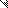# MINLPLib

### A Library of Mixed-Integer and Continuous Nonlinear Programming Instances

#### Instance ex7_2_4

 Formatsⓘ ams gms mod nl osil py Primal Bounds (infeas ≤ 1e-08)ⓘ 3.91801023 p1 ( gdx sol ) (infeas: 3e-10) Other points (infeas > 1e-08)ⓘ Dual Boundsⓘ 3.91800892 (ANTIGONE)3.91800659 (BARON)3.91801023 (COUENNE)3.91800957 (LINDO)3.91800609 (SCIP) Referencesⓘ Floudas, C A, Pardalos, Panos M, Adjiman, C S, Esposito, W R, Gumus, Zeynep H, Harding, S T, Klepeis, John L, Meyer, Clifford A, and Schweiger, C A, Handbook of Test Problems in Local and Global Optimization, Kluwer Academic Publishers, 1999.Dembo, R S, A Set of Geometric Programming Test Problems and Their Solutions, Mathematical Programming, 10:1, 1976, 192-213. Sourceⓘ Test Problem ex7.2.4 of Chapter 7 of Floudas e.a. handbook Added to libraryⓘ 31 Jul 2001 Problem typeⓘ NLP #Variablesⓘ 8 #Binary Variablesⓘ 0 #Integer Variablesⓘ 0 #Nonlinear Variablesⓘ 8 #Nonlinear Binary Variablesⓘ 0 #Nonlinear Integer Variablesⓘ 0 Objective Senseⓘ min Objective typeⓘ signomial Objective curvatureⓘ indefinite #Nonzeros in Objectiveⓘ 4 #Nonlinear Nonzeros in Objectiveⓘ 4 #Constraintsⓘ 4 #Linear Constraintsⓘ 0 #Quadratic Constraintsⓘ 2 #Polynomial Constraintsⓘ 0 #Signomial Constraintsⓘ 2 #General Nonlinear Constraintsⓘ 0 Operands in Gen. Nonlin. Functionsⓘ Constraints curvatureⓘ indefinite #Nonzeros in Jacobianⓘ 13 #Nonlinear Nonzeros in Jacobianⓘ 10 #Nonzeros in (Upper-Left) Hessian of Lagrangianⓘ 24 #Nonzeros in Diagonal of Hessian of Lagrangianⓘ 8 #Blocks in Hessian of Lagrangianⓘ 2 Minimal blocksize in Hessian of Lagrangianⓘ 4 Maximal blocksize in Hessian of Lagrangianⓘ 4 Average blocksize in Hessian of Lagrangianⓘ 4.0 #Semicontinuitiesⓘ 0 #Nonlinear Semicontinuitiesⓘ 0 #SOS type 1ⓘ 0 #SOS type 2ⓘ 0 Minimal coefficientⓘ 5.8800e-02 Maximal coefficientⓘ 4.0000e+00 Infeasibility of initial pointⓘ 105.7 Sparsity JacobianⓘSparsity Hessian of Lagrangianⓘ```\$offlisting
*
*  Equation counts
*      Total        E        G        L        N        X        C        B
*          5        1        0        4        0        0        0        0
*
*  Variable counts
*                   x        b        i      s1s      s2s       sc       si
*      Total     cont   binary  integer     sos1     sos2    scont     sint
*          9        9        0        0        0        0        0        0
*  FX      0
*
*  Nonzero counts
*      Total    const       NL      DLL
*         18        4       14        0
*
*  Solve m using NLP minimizing objvar;

Variables  x1,x2,x3,x4,x5,x6,x7,x8,objvar;

Equations  e1,e2,e3,e4,e5;

e1.. -(0.4*x1**0.67/x7**0.67 + 0.4*x2**0.67/x8**0.67 - x1 - x2) + objvar =E= 10
;

e2.. 0.0588*x5*x7 + 0.1*x1 =L= 1;

e3.. 0.0588*x6*x8 + 0.1*x1 + 0.1*x2 =L= 1;

e4.. 4*x3/x5 + 2/(x3**0.71*x5) + 0.0588*x7/x3**1.3 =L= 1;

e5.. 4*x4/x6 + 2/(x4**0.71*x6) + 0.0588*x4**1.3*x8 =L= 1;

* set non-default bounds
x1.lo = 0.1; x1.up = 10;
x2.lo = 0.1; x2.up = 10;
x3.lo = 0.1; x3.up = 10;
x4.lo = 0.1; x4.up = 10;
x5.lo = 0.1; x5.up = 10;
x6.lo = 0.1; x6.up = 10;
x7.lo = 0.1; x7.up = 10;
x8.lo = 0.1; x8.up = 10;

Model m / all /;

m.limrow=0; m.limcol=0;
m.tolproj=0.0;

\$if NOT '%gams.u1%' == '' \$include '%gams.u1%'

\$if not set NLP \$set NLP NLP
Solve m using %NLP% minimizing objvar;

```

Last updated: 2023-08-16 Git hash: 2519540e